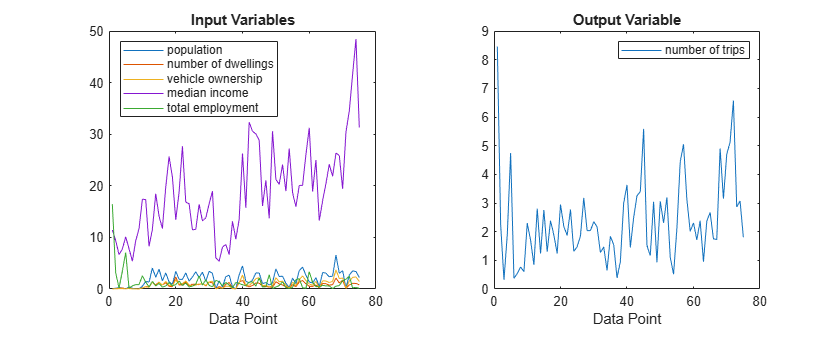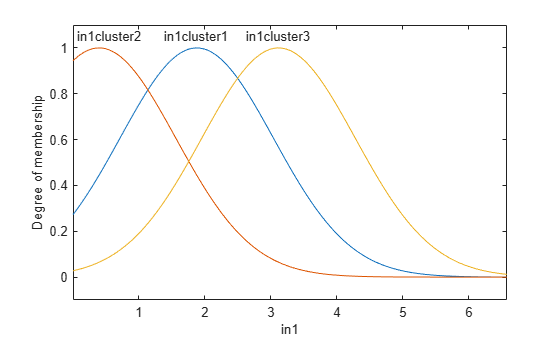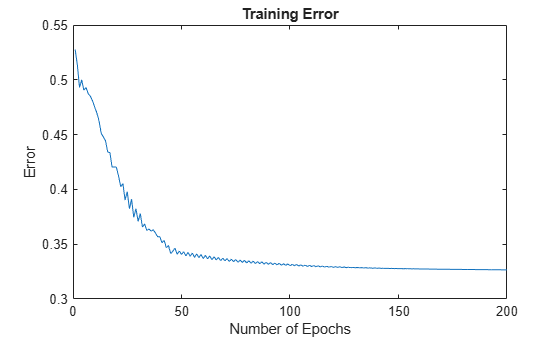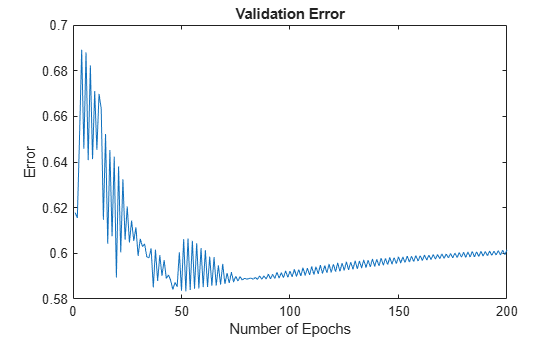Model Suburban Commuting Using Subtractive Clustering and ANFIS

This example shows how to model the relationship between the number of automobile trips generated from an area and the demographics of the area using subtractive clustering and ANFIS tuning.

This example uses demographic and trip data from 100 traffic analysis zones in New Castle County, Delaware. The data set contains five demographic factors as input variables: population, number of dwelling units, vehicle ownership, median household income, and total employment. The data contains one output variable, number of automobile trips.

Load the training and validation data.

The data set contains 100 data points, 75 for training and 25 for validation. The training input data (datain) and validation input data (valdatain) each have five columns that represent the input variables. The training output data (dataout) and validation output data (valdataout) each have one column that represents the output variable.

Plot the training data.

subplot(2,1,1)
plot(datain)
legend("population","number of dwellings","vehicle ownership",...
"median income","total employment","Location","northwest")
title('Input Variables')
xlabel('Data Point')

subplot(2,1,2)
plot(dataout)
legend("number of trips")
title("Output Variable")
xlabel('Data Point')Cluster Data

Subtractive clustering is a fast one-pass algorithm for estimating the number of clusters and the cluster centers in a data set. To cluster the training data using subtractive clustering, use the subclust function.

[C,S] = subclust([datain dataout],0.5);

For this example, use a cluster influence range of 0.5. This value indicates the range of influence of a cluster when you consider the data space as a unit hypercube. Specifying a small cluster radius usually generates many small clusters in the data, which produces a FIS with many rules. Specifying a large cluster radius usually produces a few large clusters in the data and results in fewer rules.

Each row of C contains the position of a cluster center identified by the clustering algorithm. In this case, the algorithm found three clusters in the data.

C
C = 3×6

1.8770    0.7630    0.9170   18.7500    1.5650    2.1830
0.3980    0.1510    0.1320    8.1590    0.6250    0.6480
3.1160    1.1930    1.4870   19.7330    0.6030    2.3850

Plot the training data along with the cluster centers for two of the input variables.

figure
plot(datain(:,5),dataout(:,1),'.',C(:,5),C(:,6),"r*")
legend("Data points","Cluster centers","Location","southeast")
xlabel("Total Employment")
ylabel("Number of Trips")
title("Data and Cluster Centers")The values in S show the range of influence of the cluster centers for each data dimension. All cluster centers have the same set of S values.

S
S = 1×6

1.1621    0.4117    0.6555    7.6139    2.8931    1.4395

Generate Fuzzy Inference System Using Data Clusters

Use the genfis function to generate a fuzzy inference system (FIS) from the data using subtractive clustering.

An important advantage of using a clustering method to find rules is that the resultant rules are more tailored to the input data than they are in a FIS generated without clustering. This tailoring reduces the total number of rules when the input data has a high dimension.

First, create a genfisOptions option set for subtractive clustering, specifying the same cluster influence range value.

fisOpt = genfisOptions("SubtractiveClustering",...
"ClusterInfluenceRange",0.5);

Generate the FIS model using the training data and the specified options.

fis = genfis(datain,dataout,fisOpt);

Based on the dimensions of the input and output training data, the generated FIS has five inputs and one output. genfis assigns default names for inputs, outputs, and membership functions.

The generated FIS object is a first-order Sugeno system with three rules.

showrule(fis,"Format","symbolic")
ans = 3x132 char array
'1. (in1==in1cluster1) & (in2==in2cluster1) & (in3==in3cluster1) & (in4==in4cluster1) & (in5==in5cluster1) => (out1=out1cluster1) (1)'
'2. (in1==in1cluster2) & (in2==in2cluster2) & (in3==in3cluster2) & (in4==in4cluster2) & (in5==in5cluster2) => (out1=out1cluster2) (1)'
'3. (in1==in1cluster3) & (in2==in2cluster3) & (in3==in3cluster3) & (in4==in4cluster3) & (in5==in5cluster3) => (out1=out1cluster3) (1)'

You can conceptualize each rule as follows: If the inputs to the FIS (population, dwelling units, num vehicles, income, and employment) strongly belong to their representative membership functions for a cluster, then the output (num of trips) must belong to the same cluster. That is, each rule succinctly maps a cluster in the input space to the same cluster in the output space.

Each input and output variable has three membership functions, which correspond to the three identified clusters. The parameters of the input and output membership functions are derived based on the cluster centers and cluster ranges of influence. As an example, plot the membership functions for the first input variable.

figure
plotmf(fis,"input",1)Evaluate Initial FIS Performance

Apply the training input values to the fuzzy system and find corresponding output values.

fuzout = evalfis(fis,datain);

Compute the root mean squared error (RMSE) of the output values of the fuzzy system compared to the expected output values.

trnRMSE = norm(fuzout-dataout)/sqrt(length(fuzout))
trnRMSE = 0.5276

Validate the performance of the fuzzy system using the validation data.

valfuzout = evalfis(fis,valdatain);
valRMSE = norm(valfuzout-valdataout)/sqrt(length(valfuzout))
valRMSE = 0.6179

Plot the output of the model against the validation data.

figure
plot(valdataout)
hold on
plot(valfuzout,"o")
hold off
ylabel("Output value")
legend("Validation data","FIS output","Location","northwest")The plot shows that the model does not predict the validation data well.

Tune FIS Using ANFIS

To improve the FIS performance, you can optimize the system using the anfis function. First, try using a relatively short training period (20 epochs) without using validation data, and then test the resulting FIS model against the validation data.

opt = anfisOptions('InitialFIS',fis,...
'EpochNumber',20,...
'InitialStepSize',0.1);
fis2 = anfis([datain dataout],opt);
ANFIS info:
Number of nodes: 44
Number of linear parameters: 18
Number of nonlinear parameters: 30
Total number of parameters: 48
Number of training data pairs: 75
Number of checking data pairs: 0
Number of fuzzy rules: 3

Start training ANFIS ...

1 	 0.527607
2 	 0.513727
3 	 0.492996
4 	 0.499985
5 	 0.490585
6 	 0.492924
Step size decreases to 0.090000 after epoch 7.
7 	 0.48733
8 	 0.485036
9 	 0.480813
Step size increases to 0.099000 after epoch 10.
10 	 0.475097
11 	 0.469759
12 	 0.462516
13 	 0.451177
Step size increases to 0.108900 after epoch 14.
14 	 0.447856
15 	 0.444357
16 	 0.433904
17 	 0.433739
Step size increases to 0.119790 after epoch 18.
18 	 0.420408
19 	 0.420512
20 	 0.420275

Designated epoch number reached. ANFIS training completed at epoch 20.

Minimal training RMSE = 0.420275

Assess the performance of the FIS on both the training data and the validation data.

fuzout2 = evalfis(fis2,datain);
trnRMSE2 = norm(fuzout2-dataout)/sqrt(length(fuzout2))
trnRMSE2 = 0.4203
valfuzout2 = evalfis(fis2,valdatain);
valRMSE2 = norm(valfuzout2-valdataout)/sqrt(length(valfuzout2))
valRMSE2 = 0.5894

The model performance shows substantial improvement with respect to the training data but only slightly with respect to the validation data. Plot the improved model output against the validation data.

figure
plot(valdataout)
hold on
plot(valfuzout,'o')
plot(valfuzout2,'x')
hold off
ylabel('Output value')
legend("Validation data",...
"Initial FIS: RMSE = " + num2str(valRMSE), ...
"Tuned FIS: RMSE = " + num2str(valRMSE2), ...
"Location","northwest")Check ANFIS Result for Overfitting

When tuning a FIS, you can detect overfitting when the validation error starts to increase while the training error continues to decrease.

To check the model for overfitting, use anfis with validation data to train the model for 200 epochs. First configure the ANFIS training options by modifying the existing anfisOptions option set. Specify the number of epochs and validation data. Since the number of training epochs is larger, suppress the display of training information in the Command Window.

opt.EpochNumber = 200;
opt.ValidationData = [valdatain valdataout];
opt.DisplayANFISInformation = 0;
opt.DisplayErrorValues = 0;
opt.DisplayStepSize = 0;
opt.DisplayFinalResults = 0;

Train the FIS.

[fis3,trnErr,stepSize,fis4,valErr] = anfis([datain dataout],opt);

Here:

• fis3 is the FIS object when the training error reaches a minimum.

• fis4 is the snapshot FIS object when the validation data error reaches a minimum.

• stepSize is a history of the training step sizes.

• trnErr is the RMSE using the training data.

• valErr is the RMSE using the validation data for each training epoch.

After the training completes, validate the model using the training and validation data.

fuzout4 = evalfis(fis4,datain);
trnRMSE4 = norm(fuzout4-dataout)/sqrt(length(fuzout4))
trnRMSE4 = 0.3393
valfuzout4 = evalfis(fis4,valdatain);
valRMSE4 = norm(valfuzout4-valdataout)/sqrt(length(valfuzout4))
valRMSE4 = 0.5834

The error with the training data is the lowest thus far, and the error with the validation data is also slightly lower than before. This result suggests possible overfitting, which occurs when you fit the fuzzy system to the training data so well that it no longer does a good job of fitting the validation data. The result is a loss of generality.

View the improved model output. Plot the model output against the validation data.

figure
plot(valdataout)
hold on
plot(valfuzout2,'o')
plot(valfuzout4,'x')
hold off
ylabel('Output value')
legend("Validation data",...
"Tuned FIS: RMSE = " + num2str(valRMSE2), ...
"Min val. error FIS: RMSE = " + num2str(valRMSE4), ...
"Location","northwest")Next, plot the training error trnErr.

figure
plot(trnErr)
title('Training Error')
xlabel('Number of Epochs')
ylabel('Error')This plot shows that the training error settles at about the 60th epoch.

Plot the validation error valErr.

figure
plot(valErr)
title('Validation Error')
xlabel('Number of Epochs')
ylabel('Error')The plot shows that the smallest value of the validation data error occurs at epoch 52. After this point, it increases slightly even as anfis continues to minimize the error against the training data. This pattern is a sign of overfitting. Depending on the specified error tolerance, plotting the validation error can also indicate the ability of the model to generalize the test data.